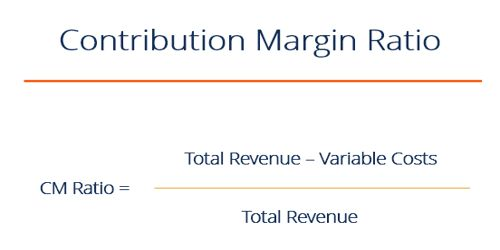# Importance of Product’s CM Ratio

The contribution margin ratio is the percentage of a firm’s contribution margin to its net sales. Contribution margin is a product’s price minus its variable costs, resulting in the incremental profit earned for each unit sold. The total contribution margin generated by an entity represents the total earnings available to pay for expenses and generate a profit.

It can be calculated as shown in the following formula:

CM Ratio = [Unit Contribution Margin / Unit Price]

= [Total Contribution Margin / Total Sales].

Importance: The contribution margin ratio is important because it shows the impact a change in sales will have on the contribution margin. For example, if a company has a contribution margin ratio of 60 percent, it means that the contribution margin increases \$0.60 for every \$1 increase in sales. Net income also increases by 60 percent if fixed costs remain the same. Companies use the contribution margin to quickly calculate how much a future increase in sales affects net income. To calculate the contribution margin that is used in the numerator in the preceding calculation, subtract all variable costs from revenues. So, the contribution margin ratio is the percentage of revenues that are available to cover a company’s fixed costs, fixed expenses, and profit. Companies can analyze which of their products yields the highest contribution margin ratio and focus on that product to increase sales. Just by calculating the contribution margin, the manager is able to promptly decide some of the more decisive points along the range of profitability.

• One main advantage of calculating the contribution margin is to know how much more you are earning in revenue than you are paying in variable costs.
• To calculate our break-even point, you need to know your contribution margin.
• Another simple but important calculation that relies upon contribution margin is net operating income or loss.
• Another use of contribution margin calculations is for the comparison of two alternative business investments.